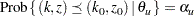Analysis after a Sequential Test

At the end of a trial, the hypothesis is either rejected or accepted. But the-value, median, and confidence limits depend on the ordering the sample space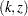, whereis the stage number andis the standardized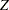statistic.

Following the notations used in Jennison and Turnbull (2000, pp. 179–180),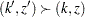if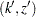has a higher order or more extreme than. Then for a given ordering, the-value, median, and confidence limits associated with the observed statisticscan be derived.

###-value

With the observed pair of statistics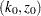when the trial is stopped, a one-sided upper-value is computed as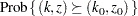A one-sided lower-value is computed as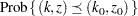A two-sided-value is twice the smaller of the lower and upper-values.

### Median Unbiased Estimate

With the observed pair, a median unbiased estimate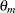is computed from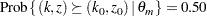### Confidence Limits

With the observed pair, a lower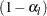confidence limit for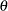,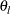, is computed from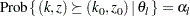Similarly, an upperconfidence limit for,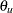, is computed from